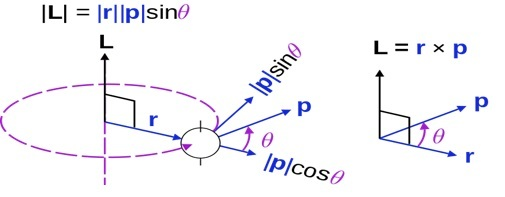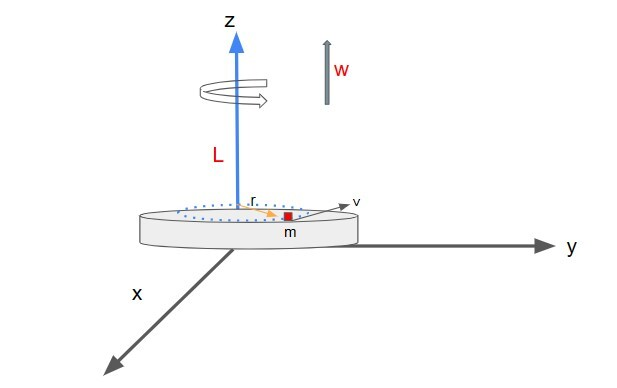# Angular Momentum - Rotation About Fixed Axis

## Introduction

Angular Momentum is a concept of rotational dynamics. We can classify motions of objects in two kinds of motions namely Translational and Rotational motions. In pure translatory motion every point of the body moves in a straight line. Each point covers the same distance in a given time interval. e.g. - A Car moving in a straight line, a man walking on the road etc. Whereas in the pure rotation, the path created by the motion of every point makes a circle whose centre lies on the axis of rotation. E.g. - earth rotating about its axis, spinning top etc.

For translational motion, in absence of external force we find the linear momentum is always conserved. We call it the principle of conservation of momentum. We used it to simplify many collision problems. One can ask, is there any such principle in Rotational motion also? The answer is-yes. In Rotational motion we have the principle of conservation of Angular momentum. Angular momentum is the angular counterpart of linear momentum. As we will see we would be able to relate it to other physical quantities like Moment of inertia, torque and linear momentum.

## Angular Momentum

Suppose a particle is rotating about an axis, then the moment linear momentum of the particle around that axis is called angular momentum of the particle.

i.e. angular momentum = linear momentum x perpendicular distance of particle

$$\mathrm{\overrightarrow{L}=\overrightarrow{p}\times \overrightarrow{r}}$$

A particle of mass m is moving with velocity v then its linear momentum L, Suppose its distance from axis of rotation is given by the radius vector $\mathrm{\overrightarrow{r}}$

### In Vector Notations Angular Momentum

$$\mathrm{\overrightarrow{L}= \overrightarrow{r}\times \overrightarrow{p}}$$

$$\mathrm{\lvert\overrightarrow{L} \rvert =rpsin\theta=mvr\:sin\theta}$$Maschen, Angular momentum definition, marked as public domain, more details on Wikimedia Commons

The dimensions of angular momentum will be $\mathrm{[ML^2 T^{-1}]}$ and SI unit is given by $\mathrm{Kg\:m^2 s^{-1}}$.

## Angular momentum in Cartesian coordinate system

We can also write the angular momentum in cartesian coordinates explicitly. First, we write the $\mathrm{\overrightarrow{r}}$ and $\mathrm{\overrightarrow{p}}$ in cartesian forms.

$$\mathrm{\overrightarrow{r}=x\hat{i}+y\hat{j}+z\hat{k}}$$

$$\mathrm{\overrightarrow{p}=p_x\hat{i}+p_y \hat{j}+p_z\hat{k}}$$

$$\mathrm{\overrightarrow{L}=(x\hat{i}+y \hat{j}+z\hat{k})\times (p_x\hat{i}+p_y\hat{j}+p_z\hat{k})}$$

$$\mathrm{\overrightarrow{L}_x=yp_z-zp_y}$$

$$\mathrm{\overrightarrow{L}_y=zp_x-xp_z}$$

$$\mathrm{\overrightarrow{L}_z=zp_y-yp_x}$$

## Derivation of Equation for Angular Momentum

First, we look at the analogous physical quantities of translational and rotational motion.

QuantityVelocityAccelerationForce MassMomentum
Translational$\mathrm{\overrightarrow{ u}}$
$\mathrm{\overrightarrow{a}}$$\mathrm{\overrightarrow{F}}$$\mathrm{m}$$\mathrm{\overrightarrow{p}} Rotational \mathrm{\overrightarrow{\omega}} (Angular velocity) \mathrm{\overrightarrow{a}} (Angular acceleration) \mathrm{\overrightarrow{\tau}}(torque) =\mathrm{\overrightarrow{r}\times \overrightarrow{F}}I(moment of inertia) = \mathrm{Σmr^2}$$\mathrm{\overrightarrow{L}}$(Angular momentum)

Table-1: Analogy between physical quantities of rotational and translational motion

We take a small particle of mass m having distance $\mathrm{\overrightarrow{r}}$ from the axis of rotation with linear momentum $\mathrm{\overrightarrow{p}}$.

From newton’s second law of motion, we have

$$\mathrm{\sum \overrightarrow{F}=\frac{d\overrightarrow{p}}{dt}}$$

We take cross product with r on both sides of this equation.

$$\mathrm{\overrightarrow{r}\times \sum \overrightarrow{F}=\overrightarrow{r}\times \frac{d\overrightarrow{p}}{dt}}$$

We recognise that

$$\mathrm{\overrightarrow{r}\times \sum \overrightarrow{F}=\overrightarrow{\tau}}$$

Hence

$$\mathrm{\overrightarrow{\tau}=\overrightarrow{r}\times \frac{d\overrightarrow{p}}{dt} ………. (1)}$$

Now let us look at this term

$$\mathrm{\frac{d\overrightarrow{r}}{dt}\times \overrightarrow{p}}$$

since

$$\mathrm{\overrightarrow{p}=m\overrightarrow{v}\:and\:\frac{d\overrightarrow{r}}{dt}=\overrightarrow{v};}$$

$$\mathrm{\overrightarrow{v}\times \overrightarrow{p}=m(\overrightarrow{v}\times \overrightarrow{v})=0}$$

(Since for any vector $\mathrm{\overrightarrow{A}:\overrightarrow{A}\times \overrightarrow{A}=0}$)

Hence, we can add it on the both sides of equation (1)

$$\mathrm{\frac{d\overrightarrow{r}}{dt}\times \overrightarrow{p}+\overrightarrow{\tau}=\overrightarrow{r}\times \frac{d\overrightarrow{p}}{dt}+\frac{d\overrightarrow{r}}{dt}\times \overrightarrow{p}=\frac{d(\overrightarrow{r}\times \overrightarrow{p})}{dt}}$$

Hence

$$\mathrm{\overrightarrow{\tau}=\frac{d(\overrightarrow{r}\times \overrightarrow{p})}{dt}}$$

If we compare it with analogous force in translational motion

$$\mathrm{\sum F=\frac{dp}{dt}}$$

we can say that term $\mathrm{(\overrightarrow{r}\times \overrightarrow{p})}$ is some kind of momentum.

Hence, we define angular momentum

$$\mathrm{\overrightarrow{L}=(\overrightarrow{r}\times \overrightarrow{p})}$$

## Angular Momentum of Rigid Body about Fixed Axis

### Angular Momentum of System

Let, there is a system of n particles, and their individual angular momenta are given by $\mathrm{\overrightarrow{l_1},\overrightarrow{l_2},\overrightarrow{l_3},............\overrightarrow{l_n}}$ then then net angular momentum of system can be written as -

$$\mathrm{\overrightarrow{L}=\overrightarrow{l_1}+\overrightarrow{l_2}+\overrightarrow{l_3}.................+\overrightarrow{l_n}=\sum_{i}\overrightarrow{L_i}}$$

$$\mathrm{\frac{d}{dt}(\overrightarrow{L})=\sum \frac{d}{dt} \overrightarrow{l_i}=\sum_i \overrightarrow{\tau_{ext,i}}}$$

Hence net external torque on a system is equal to rate of change of Total angular momentum of system.

### For Rigids Bodies

We take the rigid body as a large system of small particles. Let the mass of each particle be $\mathrm{m_i}$ and linear velocity be $\mathrm{\overrightarrow{v_i}}$.Suppose the body is moving about the z axis with angular velocity $\mathrm{\overrightarrow{\omega_i}}$ and the particles are rotating around the z axis on the x-y plane.

Then the angular momentum of each particle

$$\mathrm{\overrightarrow{L_i}=m_i(\overrightarrow{r_i}\times \overrightarrow{v_i})}$$As we can see in the figure, the angle between $\mathrm{\overrightarrow{r_i}}$ and $\mathrm{\overrightarrow{v_i}}$ is 90°.

$$\mathrm{\Rightarrow\:\:\overrightarrow{L _i}=m_i(\overrightarrow{r_i}\times \overrightarrow{ u_i})= m_i\:r_i\:v_i\: sin90=m_i\:r_i\:v_i}$$

Thus

$$\mathrm{\overrightarrow{L _z}=\sum \overrightarrow{L _i}=\sum m_i\:r_i\:v_i}$$

we know that

$$\mathrm{ u_i=\overrightarrow{r_i\omega}}$$

So

$$\mathrm{\overrightarrow{L_z}=\omega\sum m_i\:r_i^2=I \overrightarrow{\omega}}$$

since moment of inertia

$$\mathrm{I =\sum m_i\:r_i^2}$$

Hence for rigid body

$$\mathrm{\overrightarrow{L_z}=I \overrightarrow{\omega}}$$

i.e. The net Angular momentum of a rigid body about the z axis is equal to the product of angular momentum and moment of inertia.

We know $\mathrm{\overrightarrow{\tau}=\frac{d\overrightarrow{L}}{dt}}$

Hence

$$\mathrm{\overrightarrow{\tau}=\frac{d}{dt}(I\overrightarrow{\omega})=I\overrightarrow{\alpha}}$$

i.e. The Net torque acting on a rigid body is product of its moment of inertia and angular acceleration.

It gives us a relation between torque and angular acceleration. It is analogous to the Newton’s 2nd law

Translational motion Rotational motion $\mathrm{\overrightarrow{F}=m\overrightarrow{a}}$ $\mathrm{\overrightarrow{p}=m\overrightarrow{u}}$ $\mathrm{\overrightarrow{\tau}=I\overrightarrow{\alpha}}$ $\mathrm{\overrightarrow{L}=I\overrightarrow{\omega}}$

Table-2: Newton’s 2nd law in Rotational and Translational Motion

## Conclusion

Angular momentum is analogous to linear momentum in rotational motion. It depends upon the distance from the axis of rotation. Angular momentum has meaning only if it is defined about specified origin. It is a vector quantity always perpendicular to the plane of $\mathrm{\overrightarrow{r}}$ and $\mathrm{\overrightarrow{p}}$ The rate of change of angular momentum gives us Torque on the particle. We can use this concept to multi-particle systems also. For a rigid body it is equal to the angular velocity times the Moment of Inertia of that body. We can also show the correspondence between rotational and translational motion as summarised in Table-1.

## FAQs

Q1. What is the condition for conservation of angular momentum?

Ans. For an isolated system Angular momentum will always be conserved if external torque is zero. i.e.,$\mathrm{\overrightarrow{\tau_{ext}}=0}$

Q2. What does angular momentum physically signify?

Ans. Physically angular momentum is a measure of the turning motion of an object and Torque is a measure of the turning effect of force.

Q3. Linear momentum of a particle in circular motion is always changing, is it true for angular momentum too?

Ans. No, for a particle is circular path $\mathrm{\overrightarrow{L}=(mvr)\hat{k}}$ Hence it doesn’t change if a particle is moving about an axis passing through its centre.

Q4.Can a non-rotating body possess angular momentum?

Ans. Yes, suppose a particle is moving along some path in a straight line and if we take the axis at some point O, if O is displaced from that straight line, then we will get non zero angular momentum. Since $\mathrm{\overrightarrow{r}\times \overrightarrow{v}}$ will be non zero.

Q5. A sphere and a cylinder of same mass are rotating about the same axis with same angular velocity, which body will have larger angular momentum?

Ans. Moment of Inertia of cylinder > Moment of Inertia of sphere of same mass.

Hence Angular momentum of cylinder > Angular momentum of Sphere

Updated on: 28-Apr-2023

83 Views